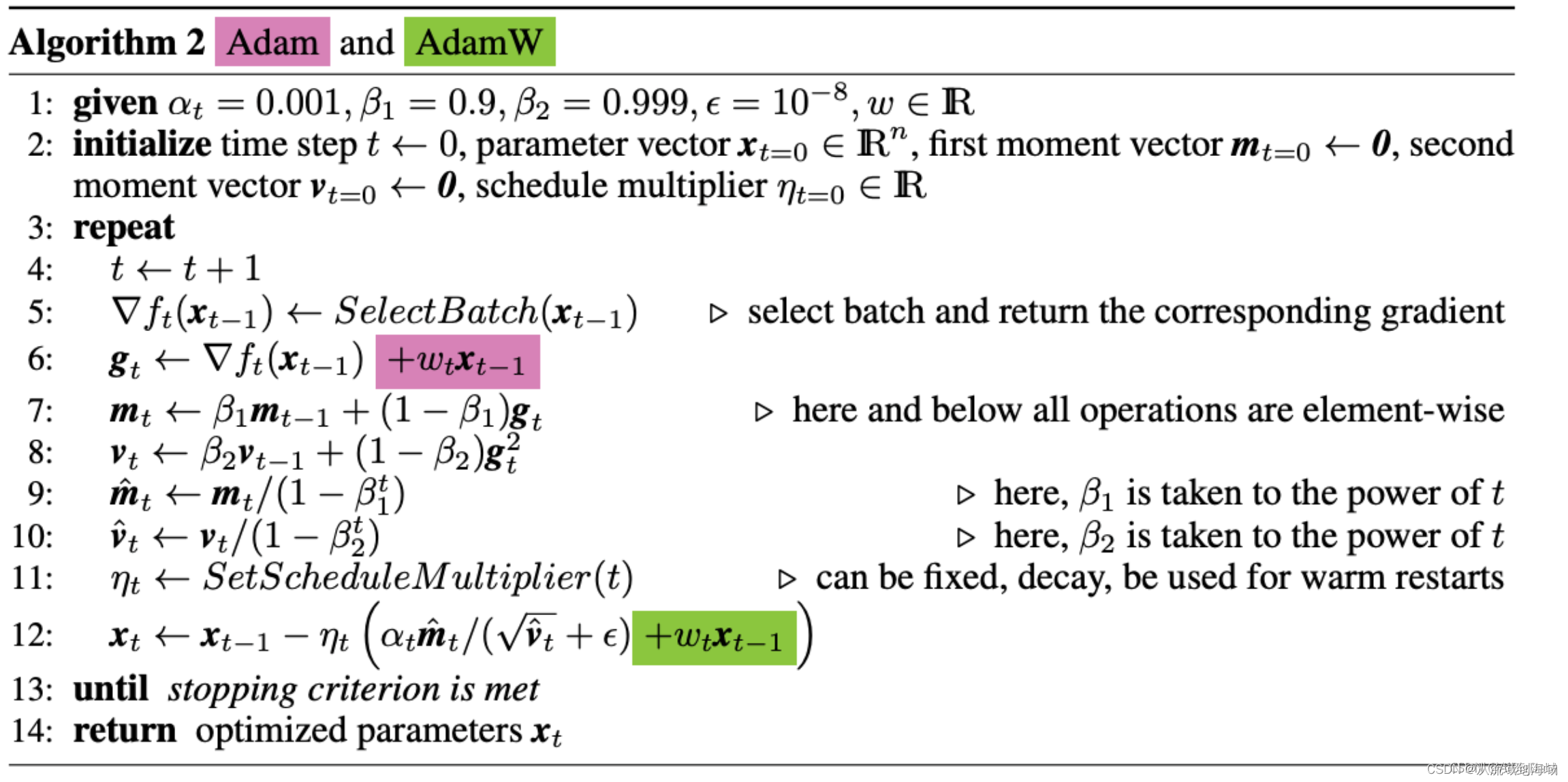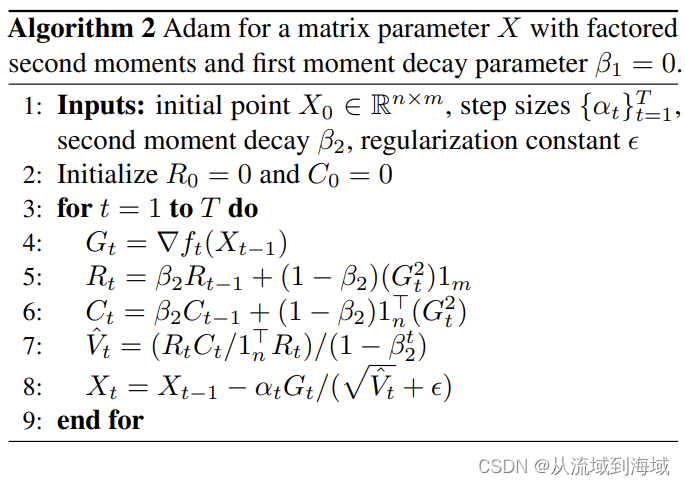##MSIPO技术圈 首页 IT技术 查看内容

2023-07-13

L ( θ n e w ) = L ( θ o l d ) + γ / 2 ∣ ∣ θ 2 ∣ ∣ （公式 1 ） L(\theta_{new})=L(\theta_{old})+\gamma/2||\theta^2|| （公式1）

g t ← ∇ f t ( θ t − 1 ) + γ θ t − 1 （公式 2 ） g_t \leftarrow \nabla f_t(\theta_{t-1}) + \gamma \theta_{t-1}（公式2）

Weight Decay即在正则项前面乘以 γ （ 0 < γ < 1 ） \gamma （0<\gamma<1） ，用来缩放正则项产生的影响：L2正则会使得参数趋近于0，Weight Decay减轻这种趋势